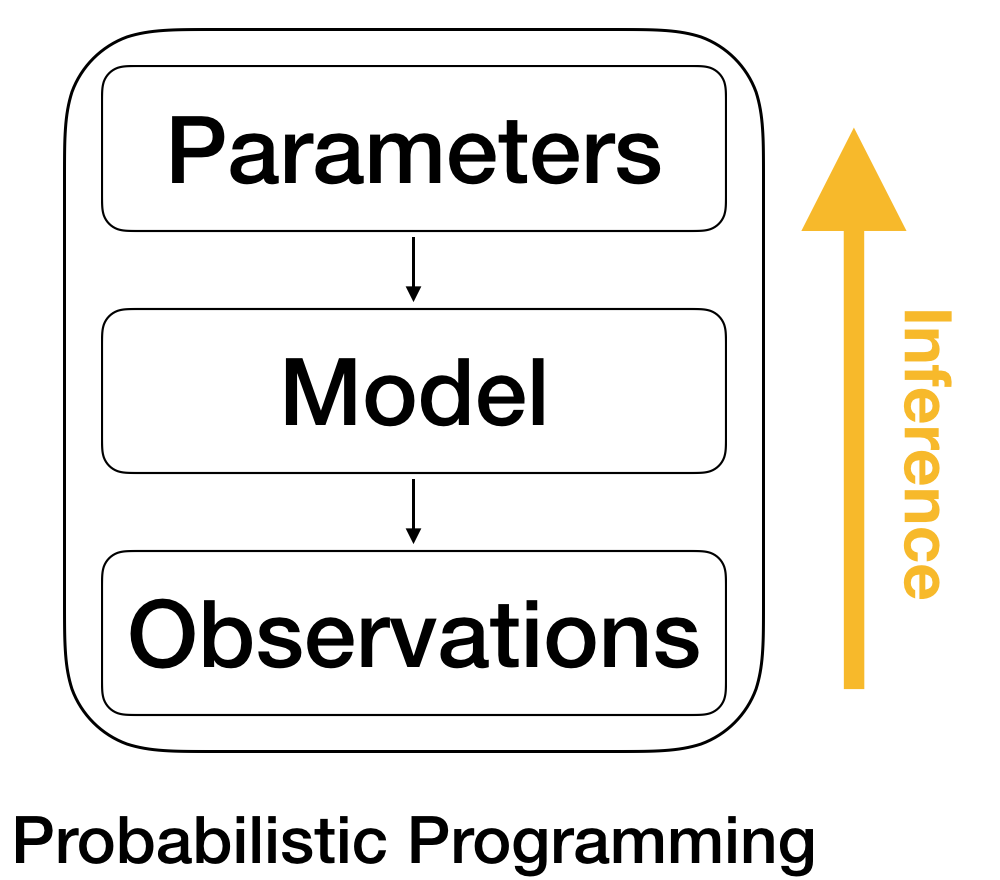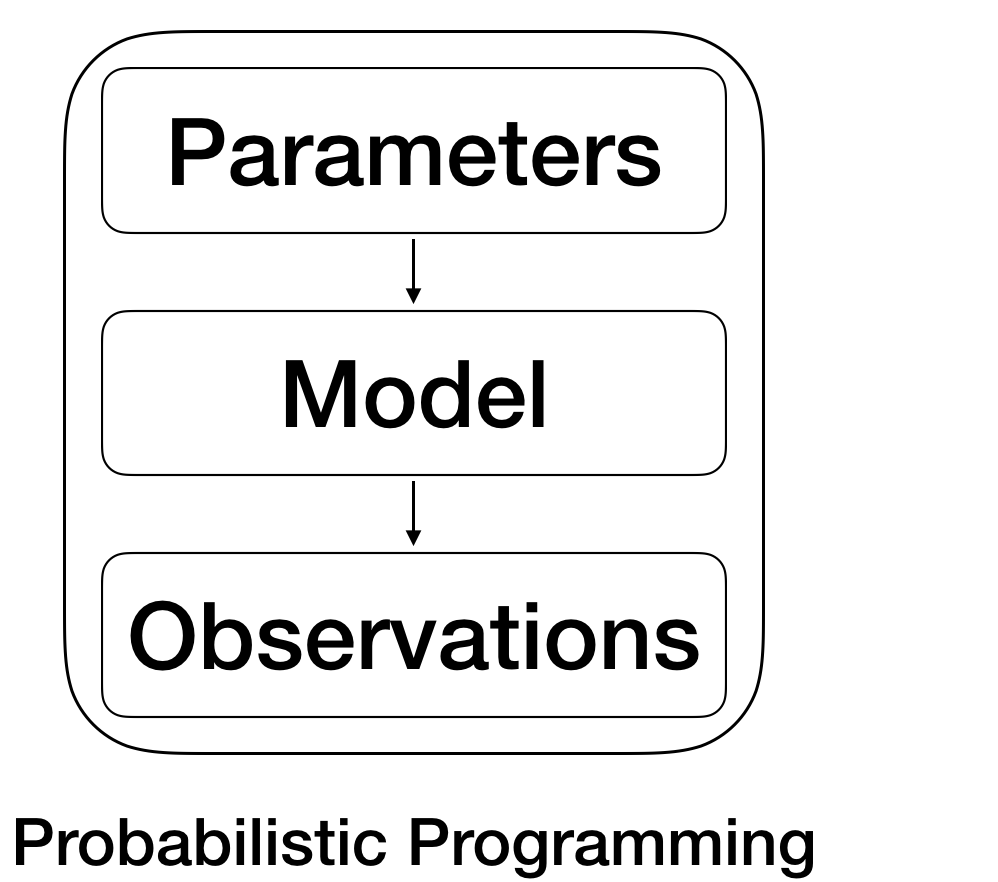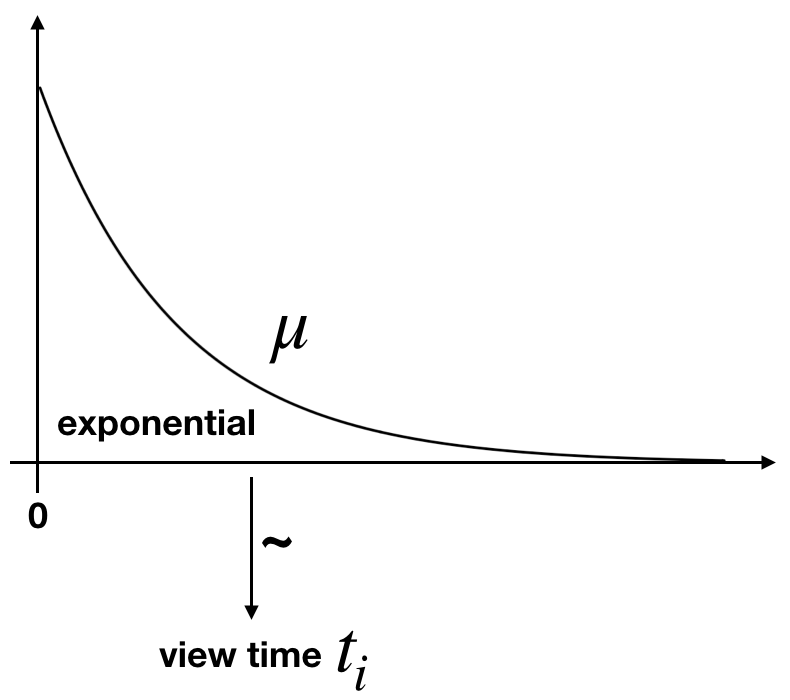# The Probabilistic Programming Workflow

### Intuition and Essentials of Model-Based Machine Learning

#### 2019-03-21

Last week, I gave a presentation about the concept of and intuition behind probabilistic programming and model-based machine learning in front of a general audience. The following are my extended notes.

The posterior probability distribution represents the unique and complete solution to the problem. There is no need to invent ‘estimators’; nor do we need to invent criteria for comparing alternative estimators with each other. Whereas orthodox statisticians offer twenty ways of solving a problem, and another twenty different criteria for deciding which of these solutions is the best, Bayesian statistics only offers one answer to a well-posed problem.

What I would like to do in the following is to show you–using a very simple example–what the typical workflow in probabilistic programming and model-based machine learning1 I am referring to the definition introduced in Winn and Bishop’s book, Model-Based Machine Learning. It provides a new view on probabilistic programming and Bayesian methods within the broader machine learning field. looks like.

I will guide you through describing our problem by defining a model, and how we can combine the latter with data to draw conclusions about the real world.

We can use the following graphic2 The graphic is very much inspired by Figure 1.2 in Jan-Willem van de Meent, Brooks Paige, Hongseok Yang and Frank Wood (2018). An Introduction to Probabilistic Programming. as the guiding thread throughout the example. But before, we discuss the graphic, let’s start with the the first step in any problem.# Step 0: Defining the Problem

The very first step is to define the problem you would like to solve. While this is very easy in the example I will present, it can be much more complicated. Yet, it is worth spending time on, because if you get this step right, it will make the following ones much easier.

Consider you’re a YouTuber, and you’re interested in the viewing behavior of your audience. Maybe you’re an educational YouTuber and upload lectures that are quite long. In particular, the videos are so long that you notice that no viewer makes it to the end of the video.3 Yes, yes, I’m only doing this because I don’t want to talk about censoring and proper survival analysis. You wouldn’t want to skip this when you define an actual problem. You decide to produce shorter videos in the future. “What’s a good length for my future videos?”, you ask yourself and open YouTube’s backend4 This is a fictional example. I don’t know what data YouTube offers its creators..

You find for every viewer i the time until he quit the video, t:

    Viewer   | Viewtime
--------------------
Viewer 1 | 10.5 min
Viewer 2 | 14.2 min
Viewer 3 | 38.0 min
...    |  ...
Viewer N |  2.8 min

We see that we have positive and continuous data, and one observation for every viewer.5 Let’s skip the possibility of returning viewers, different videos, etc. for this example. Knowing that we have this data available, we would like to answer questions, as for example:

• What is the expected time until a viewer quits?
• What’s the probability that a viewer watches longer than 10 minutes?

# InterludeHaving defined the problem setting and the available data, we are able to start the actual workflow.

We start off by following the column in the downward direction, which represents the so-called data generating process: Knowing what our data will look like, we can think about what process might have lead to the data. Specifically, what could our model and the model’s parameters look like?

Let me get into more details on how this works.

# Step 1: The Data Generating Process

## Adding the First DistributionFrom our problem setting we know that our viewers’ view times are positive and continuous, and we assume that they all quit the video before it ends. To model this data, we need a probability distribution that can generate such data. One distribution that fulfills the assumptions is the Exponential distribution. The Exponential distribution can generate data that follows our assumptions.

The Exponential distribution is defined by a single parameter, $$\mu$$. $$\mu$$ defines what data are more likely or less likely to be generated. A larger value of $$\mu$$ tends to generate longer view times $$t_i$$, while a smaller value of $$\mu$$ implies shorter view times $$t_i$$.

What we need to do in this step is to provide an initial idea of what a reasonable value for $$\mu$$ might be. This in turn defines what data $$t_i$$ we expect to be reasonable.

Importantly, this step happens before we look at our data.